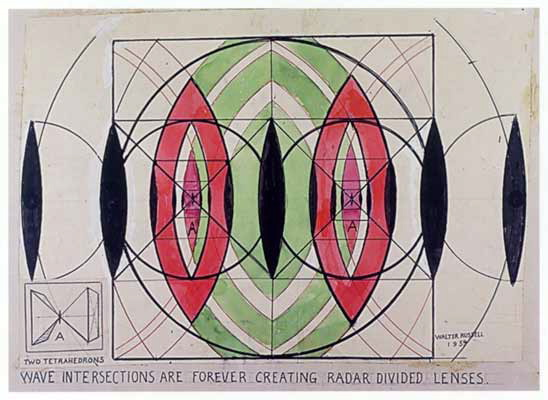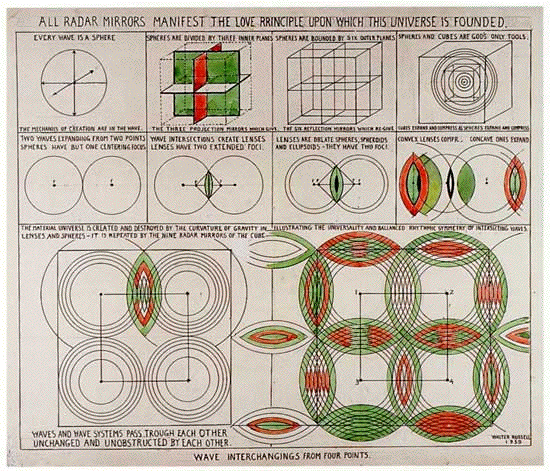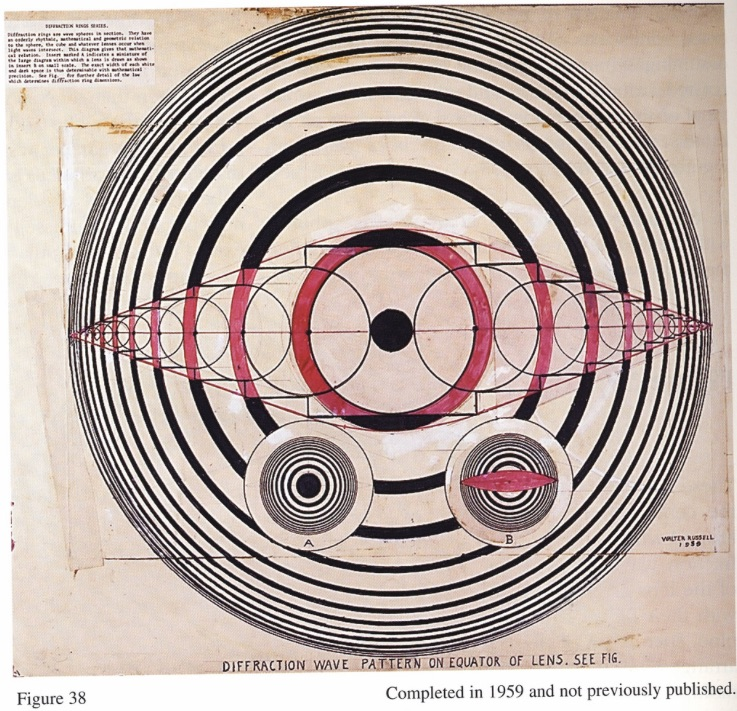# Lens

Virtual lens formed by coincidence of propagating and contracting isochronous waves. A lens is a 4++ point along the line of transmissive or propagating energy.Figure 3.4 - Focalizing Lenses at nested Cube faces.Figure 14.07 - Love Principle: Two sympathetic waves expanding from two points have one coincident centering locus.

Diffraction rings are wave spheres in sections. They have an orderly rhythmic, mathematical and geometric relation to the sphere, the cube and whatever lenses occur when light waves interact. This diagram gives that mathematical relation. Sphere marked A indicates a miniature of the large diagram within which a lens is drawn as shown in sphere B as small scale. The exact width of each white and dark space is thus determinable with mathematical precision.” [Dr. Walter Russell](courtesy University of Science and Philosophy)
(click to enlarge)